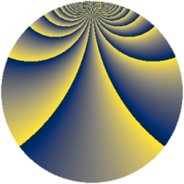# Properties

 Label 2366.2.boLevel $2366$ Weight $2$ Character orbit 2366.bo Rep. character $\chi_{2366}(121,\cdot)$ Character field $\Q(\zeta_{78})$ Dimension $2928$ Sturm bound $728$

# Related objects

## Defining parameters

 Level: $$N$$ $$=$$ $$2366 = 2 \cdot 7 \cdot 13^{2}$$ Weight: $$k$$ $$=$$ $$2$$ Character orbit: $$[\chi]$$ $$=$$ 2366.bo (of order $$78$$ and degree $$24$$) Character conductor: $$\operatorname{cond}(\chi)$$ $$=$$ $$1183$$ Character field: $$\Q(\zeta_{78})$$ Sturm bound: $$728$$

## Dimensions

The following table gives the dimensions of various subspaces of $$M_{2}(2366, [\chi])$$.

Total New Old
Modular forms 8832 2928 5904
Cusp forms 8640 2928 5712
Eisenstein series 192 0 192

## Trace form

 $$2928q + 4q^{3} - 122q^{4} + 2q^{7} - 256q^{9} + O(q^{10})$$ $$2928q + 4q^{3} - 122q^{4} + 2q^{7} - 256q^{9} - 8q^{10} + 2q^{12} + 72q^{13} + 2q^{14} + 64q^{15} + 122q^{16} + 8q^{17} + 24q^{18} - 14q^{21} + 4q^{23} - 126q^{25} + 6q^{26} + 16q^{27} + 4q^{28} - 4q^{29} - 44q^{30} - 6q^{31} + 30q^{35} - 128q^{36} + 36q^{37} + 12q^{38} - 26q^{39} - 4q^{40} - 18q^{41} - 2q^{42} - 4q^{43} + 6q^{44} + 60q^{45} - 12q^{46} + 18q^{47} - 2q^{48} + 30q^{49} + 12q^{50} + 8q^{51} - 206q^{53} - 60q^{54} + 6q^{55} - 2q^{56} - 104q^{58} + 12q^{60} + 20q^{61} + 22q^{62} + 196q^{63} + 244q^{64} + 18q^{65} - 32q^{66} - 156q^{67} - 8q^{68} + 26q^{69} + 264q^{70} + 174q^{71} - 72q^{73} + 196q^{74} - 584q^{75} - 50q^{76} - 22q^{77} - 20q^{78} - 38q^{79} - 260q^{81} - 32q^{82} - 16q^{84} - 92q^{85} - 114q^{86} + 32q^{87} + 18q^{89} - 40q^{90} - 8q^{91} + 8q^{92} + 278q^{93} + 28q^{94} + 108q^{95} - 12q^{98} + O(q^{100})$$

## Decomposition of $$S_{2}^{\mathrm{new}}(2366, [\chi])$$ into newform subspaces

The newforms in this space have not yet been added to the LMFDB.

## Decomposition of $$S_{2}^{\mathrm{old}}(2366, [\chi])$$ into lower level spaces

$$S_{2}^{\mathrm{old}}(2366, [\chi]) \cong$$ $$S_{2}^{\mathrm{new}}(1183, [\chi])$$$$^{\oplus 2}$$